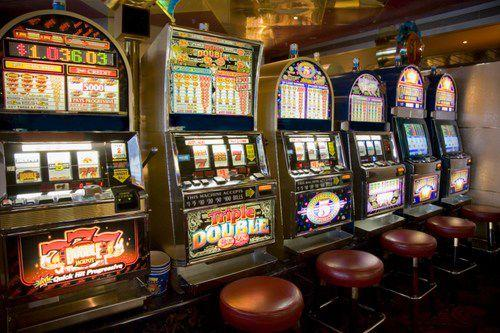## 目录

1. 强化学习介绍
1.1 学习模式
1.2 形式化为MDP问题
2. 多臂老虎机问题(Multi-Armed Bandit,MAB)
3. 探索利用困境(Exploration-exploitation dilemma)
4. 使用四种策略解决MAB问题
4.1 随机策略
4.2 ε-greedy
4.3 Boltzmann
4.4 UCB
5. 各策略的稳定性对比
6. 总结

## 1.2 形式化为MDP问题

• 表示状态空间，是描述环境的状态，表示为• 表示动作空间，是智能体可执行的动作，表示为• 表示状态之间的转移概率，状态 转移到状态s′的概率记为• 表示奖励，是环境根据智能体的动作反馈的奖励，记为## 2.多臂老虎机问题(Multi-Armed Bandit,MAB)

• 老虎机有K个摇臂，每个摇臂以一定的概率吐出金币，且概率是未知的
• 玩家每次只能从K个摇臂中选择其中一个，且相邻两次选择或奖励没有任何关系
• 玩家的目的是通过一定的策略使自己的奖励最大，即得到更多的金币

• 没有状态 ，但可以假定有一个恒定的状态• 个摇臂对应 个不同的动作• 不具有状态转移概率 ，但可以认为状态永远从转移
• 具有一个取决于 ，不取决于 的奖励函数

# 设摇臂个数为5，编号为0到4
k = 5
# 每个摇臂的真实奖励概率
real_reward = [0.2, 0.4, 0.7, 0.5, 0.3]


## 3.探索利用困境(Exploration-exploitation dilemma)

• 在强化学习中如果每个动作对应的奖励是一个确定的值，那每个动作只需尝试一次就可以知道奖励最大的动作；
• 但现实中每个动作的奖励不是确定的，每个动作的奖励通常是一个概率分布，仅一次尝试并不能真实的了解动作的奖励；
• 因此合理的做法是需要对每个动作进行多次的尝试，最终得出每个动作平均的奖励。

• 仅探索策略：所有尝试机会平均分配给每个动作，得到每个动作奖励的近似估计。
• 仅利用策略：只考虑目前奖励最大的动作。

• 探索：在自己没去过的餐馆中选择一家进行就餐，这就是探索策略
• 利用：去自己去过的三家中最好的那家餐馆，这便是利用策略

## 4.1 随机选择

import numpy as np
import pandas as pd

def random_select(N):# N为游戏次数
# 初始化各摇臂期望奖励估计
expect_reward_estimate =  * k
# 初始化各摇臂操作次数
operation =  * k
# 初始化总奖励
total_reward = 0
for i in range(N):
# 随机选择一个摇臂进行操作
arm = np.random.choice(k , size=1)
# 收集反馈的奖励数据
arm_reward = np.random.binomial(1, real_reward[arm], size=1)
# 更新期望奖励估计
expect_reward_estimate[arm] = (expect_reward_estimate[arm] * operation[arm] + arm_reward)/(operation[arm] + 1)
# 更新摇臂操作次数
operation[arm] += 1
# 更新累积奖励
total_reward += arm_reward


N = 1000
total_reward,expect_reward,operation_times = random_select(N)


print("随机选择的累积奖励：", total_reward)


# 期望奖励估计表
expect_reward_table = pd.DataFrame({
'期望奖励':expect_reward,
'操作次数':operation_times
})
expect_reward_table


## 4.2 ε-greedy

# 设定探索率
explorate_rate = 0.1
def epsilon_greedy(N, explorate_rate): # N为游戏次数
# 初始化各摇臂期望奖励估计
expect_reward_estimate =  * k
# 初始化各摇臂操作次数
operation =  * k
# 初始化总奖励
total_reward = 0
for i in range(N):
# 产生一个服从0到1之间均匀分布的随机数
r = np.random.uniform(size=1)
# 选择“利用”
if r > explorate_rate:
# 选择当前最大期望奖励所对应的摇臂进行操作
best_arm =  expect_reward_estimate.index(max(expect_reward_estimate))
# 选择“探索”
else:
# 随机选择摇臂进行操作
best_arm = np.random.choice(k, size=1)
# 收集反馈的奖励数据
best_arm_reward = np.random.binomial(1, real_reward[best_arm], size=1)
# 更新期望奖励估计
expect_reward_estimate[best_arm] = (expect_reward_estimate[best_arm] * operation[best_arm] + best_arm_reward)/(operation[best_arm] + 1)
# 更新摇臂操作次数
operation[best_arm] += 1
# 更新累积奖励
total_reward += best_arm_reward


N = 1000
total_reward,expect_reward,operation_times = epsilon_greedy(N, explorate_rate)
print("ε-greedy策略的累积奖励：", total_reward)


ε-greedy策略的累积奖励： 620

# 期望奖励估计表
expect_reward_table = pd.DataFrame({
'期望奖励':expect_reward,
'操作次数':operation_times
})
expect_reward_table


import matplotlib.pyplot as plt
plt.rcParams['font.sans-serif'] = ['SimHei']
# 在0到1之间生成100个探索率
# 在不同探索率下，ε-greedy策略的累积奖励
reward_result = [epsilon_greedy(N, i) for i in explore_grad]
# 绘制折线图
plt.figure(figsize=(8, 6))
plt.xlabel('探索率',fontsize=12)
plt.ylabel('累积奖励',fontsize=12)
plt.xlim(0,1)
plt.show()


## 4.3 Boltzmann

ε-greedy策略并不是一个高效的算法，它基于“先训练再测试”的设定，只要摇臂被认为不是“当前最佳”的摇臂，在它们上面分配的次数就是一样多的。然后我们尝试Boltzmann策略，它的核心思想是不应该根据摇臂能够带来多大的收益来对每一个摇臂分配实验次数，而应该根据它“有多大可能是最佳的摇臂”，Boltzmann探索策略以归一化后的概率操作第 个摇臂，它是根据Boltzmann分布进行摇臂的选择：# sigma是Boltzmann的参数
sigma = 0.1

def boltzmann(N, sigma):
# 初始化各摇臂期望奖励估计
expect_reward_estimate =  * k
# 初始化各摇臂操作次数
operation =  * k
# 初始化总奖励
total_reward = 0
for i in range(N):
# 通过Boltzmann分布计算摇臂的奖励概率
reward_prob = np.exp(np.array(expect_reward_estimate)/sigma)/np.exp(np.array(expect_reward_estimate)/sigma).sum()
# 通过奖励概率的分布进行抽样，选择摇臂
best_arm = np.random.choice(k, size=1, p=reward_prob)
# 收集反馈的奖励数据
best_arm_reward = np.random.binomial(1, real_reward[best_arm], size=1)

# 更新期望奖励估计
expect_reward_estimate[best_arm] = (expect_reward_estimate[best_arm] * operation[best_arm] + best_arm_reward)/(operation[best_arm] + 1)
# 更新摇臂操作次数
operation[best_arm] += 1
# 更新累积奖励
total_reward += best_arm_reward


N = 1000
total_reward,expect_reward,operation_times = boltzmann(N,sigma)
print("Boltzmann策略的累积奖励：", total_reward)


Boltzmann策略的累积奖励： 690

# 期望奖励估计表
expect_reward_table = pd.DataFrame({
'期望奖励':expect_reward,
'操作次数':operation_times
})
expect_reward_table


# 在0到1之间生成100个sigma
# 得到在这100个sigma下，Boltzmann策略的累积奖励
reward_result = [boltzmann(N, i) for i in sigma_grad]
# 绘制折线图
plt.figure(figsize=(8, 6))
plt.xlabel('探索率', fontsize=12)
plt.ylabel('累积奖励', fontsize=12)
plt.xlim(0, 1)
plt.show()


## 4.4 UCBdef ucb(N):
# 初始化各摇臂期望奖励估计
expect_reward_estimate =  * k
# 初始化各摇臂操作次数
operation =  * k
# 初始化总奖励
total_reward = 0
for i in range(N):
# 初始化最大置信区间上限
max_upper_bound = 0
# 遍历所有摇臂，根据UCB值选择最佳摇臂
for j in range(k):
if (operation[j] > 0):
# 计算探索程度的大小
delta_i = np.sqrt(2 * np.log(i + 1)/operation[j])
# 计算UCB值
upper_bound = expect_reward_estimate[j] + delta_i
else:
# 初始化UCB值
upper_bound = 1e400
if upper_bound > max_upper_bound:
# 更新UCB值
max_upper_bound = upper_bound
# 选择最佳摇臂
best_arm = j
# 收集反馈的奖励数据
best_arm_reward = np.random.binomial(1, real_reward[best_arm], size=1)
# 更新期望奖励估计
expect_reward_estimate[best_arm] = (expect_reward_estimate[best_arm] * operation[best_arm] + best_arm_reward)/(operation[best_arm] + 1)
# 更新摇臂操作次数
operation[best_arm] += 1
# 更新累积奖励
total_reward += best_arm_reward


N = 1000
total_reward,expect_reward,operation_times = ucb(N)
print("UCB策略的累积奖励：", total_reward)


UCB策略的累积奖励： 623

# 期望奖励估计表
expect_reward_table = pd.DataFrame({
'期望奖励':expect_reward,
'操作次数':operation_times
})
expect_reward_table


## 5. 各策略的稳定性对比

# 进行模拟
random_select_simulate = [random_select(N) for i in range(100)]
epsilon_greedy_simulate = [epsilon_greedy(N, 0.1) for i in range(100)]
boltzmann_simulate = [boltzmann(N, 0.1) for i in range(100)]
ucb_simulate = [ucb(N) for i in range(100)]

# 计算平均累积奖励
print('随机选择策略的平均累积奖励：', np.array(random_select_simulate).mean())
print('ε-greedy策略的平均累积奖励：', np.array(epsilon_greedy_simulate).mean())
print('Boltzmann策略的平均累积奖励：', np.array(boltzmann_simulate).mean())
print('UCB策略的平均累积奖励：', np.array(ucb_simulate).mean())


ε-greedy策略的平均累积奖励： 638.3
Boltzmann策略的平均累积奖励： 647.25
UCB策略的平均累积奖励： 617.39

# 绘制折线图
plt.figure(figsize=(10, 6))
plt.title('四种策略的对比',fontsize=18)
plt.plot(range(100), random_select_simulate, c='royalblue', label='random select') # 随机策略
plt.plot(range(100), epsilon_greedy_simulate, c='red', label='ε-greedy') # ε-greedy策略
plt.plot(range(100), boltzmann_simulate, c='teal', label='Boltzmann') # Boltzmann策略
plt.plot(range(100), ucb_simulate, c='g', label='UCB') # UCB策略
plt.xlabel('模拟次数', fontsize=18)
plt.ylabel('累积奖励', fontsize=18)
plt.grid(True)
plt.ylim(300, 750)
plt.legend(loc='lower right',fontsize=12)
plt.show()